chp5-4.mw

Consider a portfolio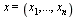such that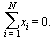Such a portfolio is self financed. The absolute percentage invested in the short position and in the long position is equal. Hence, the amount invested in long positions equals that in short positions and thus such a portfolio does not require out of pocket financing.

The arbitrage in this model is in terms of risk and (expected) rate of return.

The premise is that if there exists securities such that their expected rate of return does not fully compensate the holder for the risk borne or over compensate for it, a riskless self financed portfolio with positive (expected) rate of return exists.  The existence of such a portfolio means that the investor in this market can have an investment instrument that requires no money while producing positive expected rate of return. Such an opportunity will be exploited by investors generating a price pressure that will increase the price of the securities that are in a long position in this portfolio and decreases the prices of the securities that are held in a short position.  Consequently prices will adjust such that the opportunity to obtain positive (expected) rate of return with zero money and no risk-arbitrage opportunities will disappear. Hence the assumption is that such a portfolio does not exist in the market. The absence of such a portfolio implies the required result.

The identification of such a portfolio can be formulated as a linear programming maximization problem. The objective function is the (expected) rate of return from the portfolio and the constraints ensure that the portfolio is riskless and self financed.  Since the optimization problem requires the portfolio to be riskless its expected rate of return is its rate of return, and thus we refer to it as (expected) rate of return. Hence the optimization problem is given by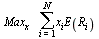s.t.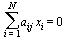for  j=1,...,s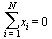In this problem we are looking for a portfolio with a risk profile of (0,...,0).

Each of the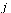constraints of the typeensures that the portfolio is riskless.

The rate of return of the portfolio is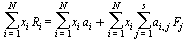which as we explained before can be written as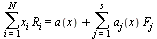where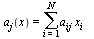andHowever, since each of the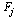s are multiplied by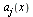which is 0, this is a non-risky portfolio (to the extent that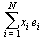is indeed to be zero). Thus its rate of return, which equals its expected rate of return since it is a riskless portfolio, is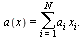Moreover, the constraintensures that the cost of this portfolio is zero.  If an optimal solution to this optimization problem with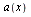not being zero exists, there exists in this market a self financed portfolio producing a positive risk free rate of return.

Let us take a second look at this optimization problem. It is obviously a linear programming problem since all the involved functions are linear. Furthermore its optimal value can either be zero or it will be unbounded. If a feasible solution for whichis positive (negative) exists scaling this solution will increasewithout bound. If the problem is unbounded it means that a portfolio whose (expected) return is infinite with no cost and bears no risk, exists in the market. Equilibrium cannot prevail in such a market and hence we assume that such a portfolio does not exist and therefore assume that the optimal value of this problem must be zero. This is the assumption made by the APT, namely the absence of arbitrage opportunities.

The feasible set of the optimization problem consists of all the vectors that are perpendicular to the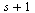vectors; the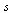dimensional vector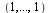and thevectors: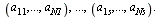As we just explained the optimal value of the objective function, if there are no arbitrage opportunities, must be zero.  However this will be the case if and only if vector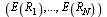must be in the subspace spanned by the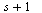vectors above. That is, there must exist an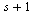dimensional vector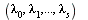such that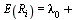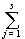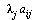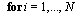One of the end of chapter questions asks the reader to prove the above statement. It is also proven at the end of this section with the aid of the Lagrangian which shows that the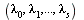are the Lagrangian multipliers of the above optimization problem.

The above equation is the multidimensional counterpart of the SML in which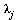plays the rule of β. The price of one unit of risk of type(captured by theth factor) is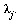To reinforce this interpretation let us see what is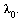If a risk free asset exists in this market, and its rate of return is R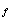then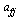=0 for everyand thus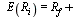.

Now it is more apparent that the rate of return on security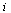is the risk free rate plus the compensation of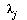per one unit of risk. That is for each unit of factorthat is embedded in securitythe compensation in terms of rate of return is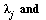hence for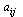units of factorthe compensation is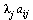and the total compensation is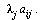Similarly we can present the other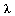in terms of portfolios that are influenced only by one factor. These are portfolios that have risk profiles presented by a vector in the neutral basis. Let us denote by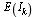the expected rate of return on a portfolio with a  risk profile of (0,...,1,0,...0) where 1 stands at the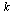th position. That is a portfolio that is sensitive only to the risk of theth factor, i.e.,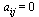for allexcept for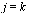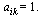Hence for such a portfolio the equation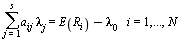implies that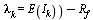.

We therefore can state that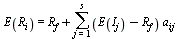for every i

which of course resembles the SML where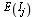plays the rule of the rate of return on the market portfolio.

The Lagrangian of the optimization problem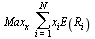s.t.for  j=1,...,sis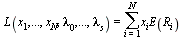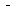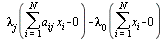In chapter 1 we investigated the first order conditions for such (convex) optimization problems. We also mentioned that the optimal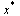and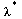correspond to a saddle point of the Lagrangian and hence that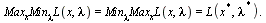It is also easy to show from first principals that the optimal value of the optimization problem above equals to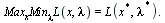Hence if no arbitrage opportunities exists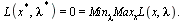The APT main result is a consequence of the first order condition ofand of the optimal value of this problem being zero.  This result is can be obtained applying virtually the same arguments (and geometric interpretation) that we used in Chapter 1 to prove the first order conditions for constrained optimization.

For a fixed λ the first order condition of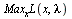are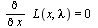which implies that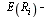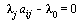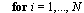Substituting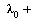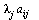for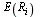in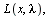indeed yields that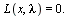Thus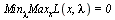if and only if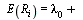and consequently there are no arbitrage opportunities in the market if and only if the above equality is satisfied.